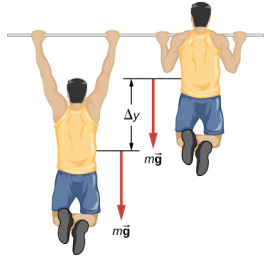$$\require{cancel}$$

# 7.5: Power

•• Contributed by OpenStax
• General Physics at OpenStax CNX

Learning Objectives

• Relate the work done during a time interval to the power delivered
• Find the power expended by a force acting on a moving body

The concept of work involves force and displacement; the work-energy theorem relates the net work done on a body to the difference in its kinetic energy, calculated between two points on its trajectory. None of these quantities or relations involves time explicitly, yet we know that the time available to accomplish a particular amount of work is frequently just as important to us as the amount itself. In the chapter-opening figure, several sprinters may have achieved the same velocity at the finish, and therefore did the same amount of work, but the winner of the race did it in the least amount of time.

We express the relation between work done and the time interval involved in doing it, by introducing the concept of power. Since work can vary as a function of time, we first define average power as the work done during a time interval, divided by the interval,

$P_{ave} = \frac{\Delta W}{\Delta t} \ldotp \label{7.10}$

Then, we can define the instantaneous power (frequently referred to as just plain power).

Definition: Power

Power is defined as the rate of doing work, or the limit of the average power for time intervals approaching zero,

$P = \frac{dW}{dt} \ldotp \label{7.11}$

If the power is constant over a time interval, the average power for that interval equals the instantaneous power, and the work done by the agent supplying the power is

$W = P \Delta t.$

If the power during an interval varies with time (i.e., $$P(t)$$), then the work done is the time integral of the power,

$W = \int P (t) dt \ldotp$

The work-energy theorem relates how work can be transformed into kinetic energy. Since there are other forms of energy as well, as we discuss in the next chapter, we can also define power as the rate of transfer of energy. Work and energy are measured in units of joules, so power is measured in units of joules per second, which has been given the SI name watts, abbreviation W: 1 J/s = 1 W. Another common unit for expressing the power capability of everyday devices is horsepower: 1 hp = 746 W.

Example $$\PageIndex{1}$$: Pull-Up Power

An 80-kg army trainee does pull-ups on a horizontal bar (Figure $$\PageIndex{1}$$). It takes the trainee 0.8 seconds to raise the body from a lower position to where the chin is above the bar. How much power do the trainee’s muscles supply moving his body from the lower position to where the chin is above the bar? (Hint: Make reasonable estimates for any quantities needed.)Figure $$\PageIndex{1}$$: What is the power expended in doing ten pull-ups in ten seconds?

Strategy

The work done against gravity, going up or down a distance $$\Delta$$y, is mg$$\Delta$$y. Let’s assume that $$\Delta$$y = 2 ft ≈ 60 cm. Also, assume that the arms comprise 10% of the body mass and are not included in the moving mass. With these assumptions, we can calculate the work done.

Solution

The result we get, applying our assumptions, is

$P=\frac{\operatorname{mg}(\Delta y)}{t}=\frac{0.9(80 \: \mathrm{kg})\left(9.8 \: \mathrm{m} / \mathrm{s}^{2}\right)(0.60 \: \mathrm{m})}{0.8 \: \mathrm{s}}=529 \: \mathrm{W}$

Significance

This is typical for power expenditure in strenuous exercise; in everyday units, it’s somewhat more than one horsepower (1 hp = 746 W).

Exercise $$\PageIndex{1}$$

Estimate the power expended by a weightlifter raising a 150-kg barbell 2 m in 3 s.

Add texts here. Do not delete this text first.

The power involved in moving a body can also be expressed in terms of the forces acting on it. If a force $$\vec{F}$$ acts on a body that is displaced d$$\vec{r}$$ in a time dt, the power expended by the force is

$P = \frac{dW}{dt} = \frac{\vec{F}\; \cdotp d \vec{r}}{dt} = \vec{F}\; \cdotp \left(\dfrac{d \vec{r}}{dt}\right) = \vec{F}\; \cdotp \vec{v}, \label{7.12}$

where $$\vec{v}$$ is the velocity of the body. The fact that the limits implied by the derivatives exist, for the motion of a real body, justifies the rearrangement of the infinitesimals.

Example $$\PageIndex{2}$$: Automotive Power Driving Uphill

How much power must an automobile engine expend to move a 1200-kg car up a 15% grade at 90 km/h (Figure $$\PageIndex{2}$$)? Assume that 25% of this power is dissipated overcoming air resistance and friction.Figure $$\PageIndex{2}$$: We want to calculate the power needed to move a car up a hill at constant speed.

Strategy

At constant velocity, there is no change in kinetic energy, so the net work done to move the car is zero. Therefore the power supplied by the engine to move the car equals the power expended against gravity and air resistance. By assumption, 75% of the power is supplied against gravity, which equals m$$\vec{g}\; \cdotp \vec{v}$$ = mgv sin $$\theta$$, where $$\theta$$ is the angle of the incline. A 15% grade means tan $$\theta$$ = 0.15. This reasoning allows us to solve for the power required.

Solution

Carrying out the suggested steps, we find

$0.75 P = mgv \sin(\tan^{−1} 0.15),$

or

$P = \frac{(1200 \times 9.8\; N)(\frac{90\; m}{3.6\; s}) \sin (8.53^{o})}{0.75} = 58\; kW,$

or about 78 hp. (You should supply the steps used to convert units.)

Significance

This is a reasonable amount of power for the engine of a small to mid-size car to supply (1 hp = 0.746 kW). Note that this is only the power expended to move the car. Much of the engine’s power goes elsewhere, for example, into waste heat. That’s why cars need radiators. Any remaining power could be used for acceleration, or to operate the car’s accessories.